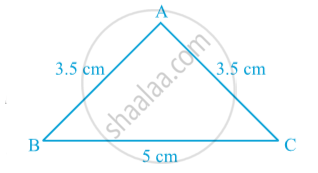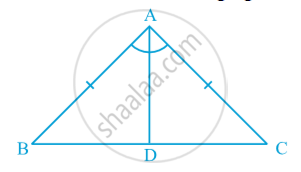Share

# Properties of a Triangle

#### notes

Construct a triangle in which two sides are equal, say each equal to 3.5 cm and the third side equal to 5 cm in following fig.A triangle in which two sides are equal is called an isosceles triangle. So, ∆ ABC of above Fig. is an isosceles triangle with AB = AC.

#### theorem

Theorem : Angles opposite to equal sides of an isosceles triangle are equal.
Proof : We are given an isosceles triangle ABC in which AB = AC. We need to prove that ∠ B = ∠ C.  Let us draw the bisector of ∠ A and let D be the point of intersection of this bisector of
∠ A and BC in following fig.AB = AC (Given)
So,  ∠ ABD = ∠ ACD, since they are corresponding angles of congruent triangles.
So,  ∠ B = ∠ C

Theorem : The sides opposite to equal angles of a triangle are equal.
This is converse of above theorem.

### Shaalaa.com

Properties of triangle [00:16:19]
S
0%

S